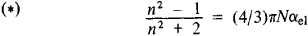# Lorentz-Lorenz Formula

## Lorentz-Lorenz Formula

a formula that relates the refractive index n of a substance to the electronic polarizability αel of the constituent particles. The formula was obtained independently by H. A. Lorentz and by the Danish physicist L. Lorenz in 1880. For a substance all of whose particles are identical, the Lorentz-Lorenz formula has the formwhere N is the number of polarizable particles per unit volume. In the case of a mixture of k substances, the right-hand side of (*) is replaced by the sum of k terms, (4/3) π Niαi el (i = 1, 2, & , k), each of which refers to only one of the substances (the sum of all Ni is equal to N).

The Lorentz-Lorenz formula was derived on the basis of assumptions that are valid only for isotropic media (gases, nonpolar liquids, cubic crystals). However, as experiment shows, (*) is also approximately satisfied for many other substances (the justification of its use and the degree of accuracy are determined experimentally in each individual case). The Lorentz-Lorenz formula is inapplicable in the regions of intrinsic (resonance) absorption bands of substances—that is, in regions of anomalous dispersion of light.

The polarizability of a substance may be considered to be purely electronic only for external field frequencies that correspond to visible and ultraviolet radiation. The Lorentz-Lorenz formula as shown in (*) is applicable only in these regions (with the limitations indicated above). For lower frequencies of the field, in the infrared region, ion cores (atoms) that are heavier than electrons can also be displaced, and their contribution to the polarizability αat must be taken into account. In many cases it is sufficient to replace aei in the formula (*) by the total “elastic” polarizability (αel and αat; it should be kept in mind that the dielectric constant is € = n2). So-called orientational polarization, which is due to the rotation of the permanent dipole moments of the particles “along the field,” is significant in polar dielectrics in the region of the spectrum with wavelengths longer than the infrared wavelengths. Taking into account this polarization makes the dependence of n on α more complicated for these frequencies (the Langevin-Debye formula).

Despite all the limitations on its applicability, the Lorentz-Lorenz formula is used extensively. The formula and the expression for molecular refraction that follows directly from it are the basis for the refractometry of pure substances and mixtures, for determining the polarizability of particles, and for investigating the structure of organic and inorganic compounds.

### REFERENCES

Landsberg, G. S. Optika, 4th ed. Moscow, 1957. (Obshchii kurs fiziki, vol. 3.)
Vol’kenshtein, M. V. Molekuliarnaia optika. Moscow-Leningrad, 1951.
Batsanov, S. S. Strukturnaia refraktometriia. Moscow, 1959.
Born, M., and E. Wolf. Osnovy optiki. Moscow, 1970. (Translated from English.)

V. A. ZUBKOV

Site: Follow: Share:
Open / Close